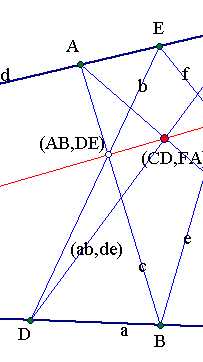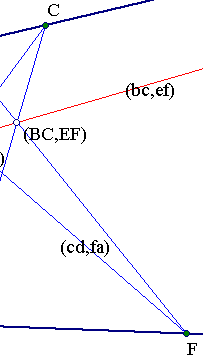##Pappus self-duality

The projectively dual of the Pappus theorem is the same as the original theorem. This is equivalent with the dual producing the same figure as the original.Pappus theorem deals with an hexagon ABCDEF inscribed in the degenerate conic of P2 (projective plane) defined by two lines (a) and (d). The vertices of the hexagon must alternatively lie on the two lines. Then the intersections of opposite sides are considered. This means taking pairs of sides (AB, DE) leaving out a letter and cyclic permuting. The two other pairs being (BC, EF) and (CD, FA).
The theorem asserts that the intersection points of these three pairs are on a line.
The dual considers an hexagon of P2* (dual projective space of lines of P2) inscribed into a degenerate conic consisting of two lines of P2*. Identify the two lines of P2* with the bundles of lines at points B and E. Taking points alternatively on these two lines generates the dual polygon abcdef. The pairs of opposite sides are again (ab, de), (bc, ef), (cd, fa). Each pair defines an intersection point denoted by the same symbol, which is a point of P2* i.e. a line of P2. The three points of P2* are on the same line of P2*, which means that the three lines of P2 pass through the same point of P2. In the above configuration this is point (CD,FA).
Another instance of the duality connecting two different figures offers the theorem of Brianchon, its dual being the theorem of Pascal.